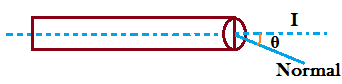# Introduction to Electric Current and Current Density

Electric Current(I): The rate of flow of electric charge through an object is called electric current.

$$\text{i.e., Current (I)} = \frac{ \text{Charge(q)}}{ \text{time(t)}}$$

If  is the amount of charge that will flow through an object in time . Then,     $$\text{ I} = \frac{ \Delta \text{ q}}{\Delta \text{ t}}$$

Instantaneous current: The current at the particular instant is called instantaneous current.

$$\text{i.e., I}\lim_{ \Delta t \to 0} \frac{ \Delta \text{ q}}{\Delta \text{ t}}$$

$$\text{or, I} = \frac{ \text{ d q}}{ \text{ d t}}$$

Current is measured in   or ampere(A) in SI unit.

1C= 3 ×109 e.s.u (electrostatic unit) of charge

1C= 1/10 e.m.u (electromagnetic unit) of charge

$$\text{1A} = \frac{\text{(1/10 e.m.u. of charge)}}{ \text{1s}}$$

$$\text{or, 1A} = \text {e.m.u. of current}$$

$$\text{or, 1A = 1/10 Biot (CGS unit of current)}$$

Current density (J): the amount of current per unit cross-section area perpendicular to the surface is called current density.

$$\text{i.e., J} = \frac{\text{I}}{ \text{A}}$$If θ be the angle between the direction of current and normal to the surface,

Then,

$$\text{J} = \frac{\text{I}}{ \text{Acos} \theta }$$

$$\text{or, I} = \vec{J} . \vec{A}$$

Current density is a vector quantity and its direction is in the direction of the conventional current.

Do you like this article ? If yes then like otherwise dislike :